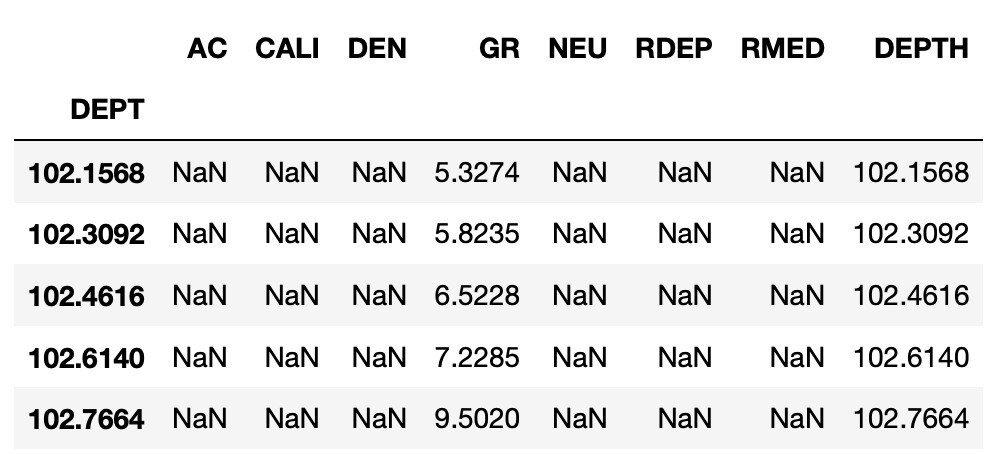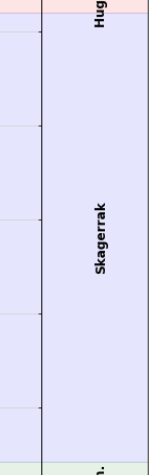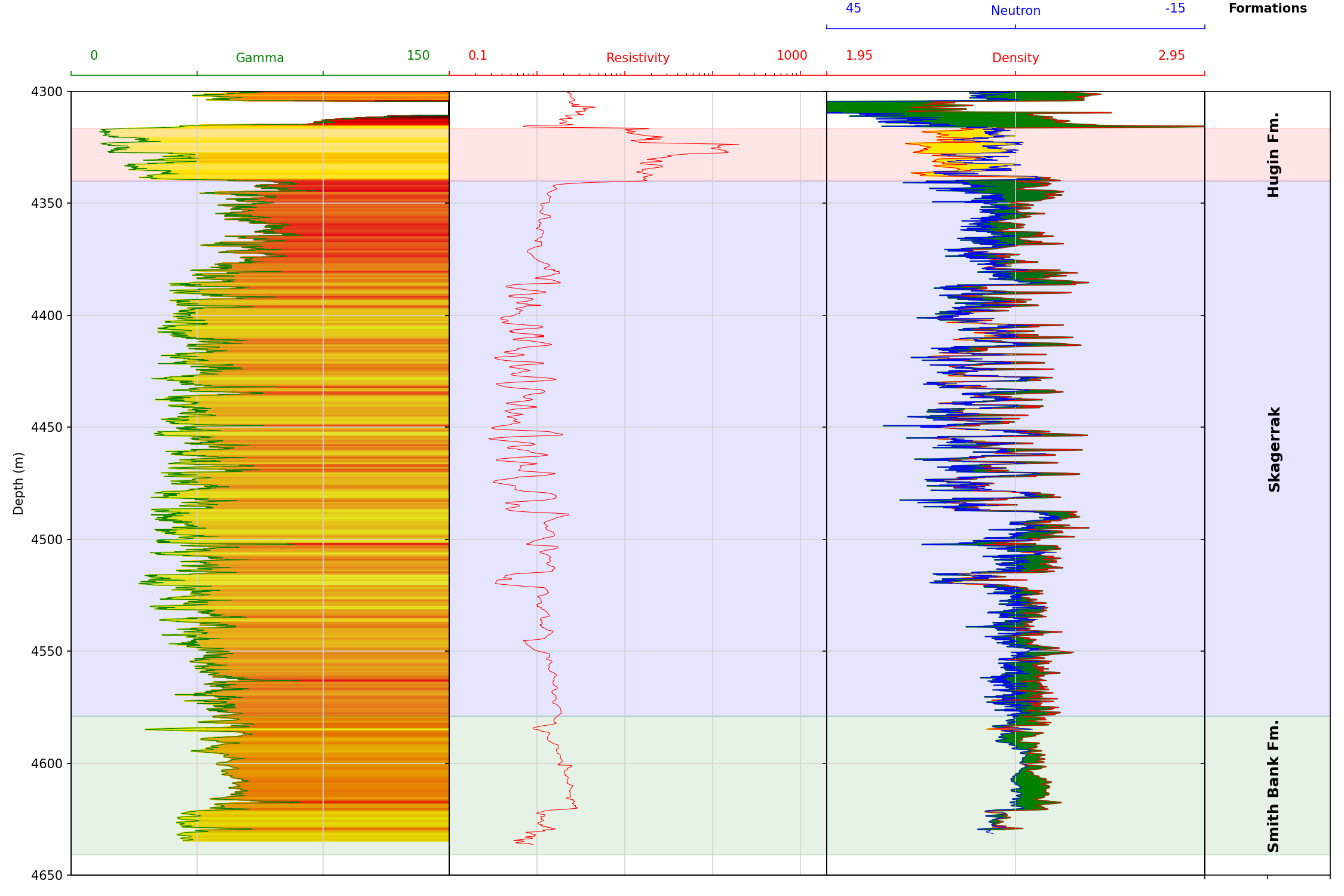## Adding Formation Data to a Well Log Plot

Well log plots are a common visualization tool within geoscience and petrophysics. They allow easy visualization of data (for example, Gamma Ray, Neutron Porosity, Bulk Density, etc) that has been acquired along the length (depth) of a wellbore.

I have previously covered different aspects of making these plots in the following articles:

In this article, I will show how to combine these different methods into a single plot function that allows you to easily reuse the code with similar data.

For the examples below you can find my Jupyter Notebook and dataset on my GitHub repository at the following link. andymcdgeo/Petrophysics-Python-Series *This series of Jupyter Notebooks take you through various aspects of working with Python and Petrophysical data. A…*github.com

This article forms part of my Python & Petrophysics series. Details of which can be found here.

The dataset we are using comes from the publicly available Equinor Volve Field dataset released in 2018. The file used in this tutorial is from well 15/9- 19SR which contains a nice set of well log data.

## Creating a Reusable Log Plot and Showing Formation Data

### Setting up the Libraries

For this article and notebook, we will be using a number of different libraries.

We will be using five libraries: pandas, matplotlib, csv, collecitons, and lasio. Pandas and lasio, will be used to load and store the log data, collections allow us to use a defaultdict to load in our formation tops to a dictionary. Finally, matplotlib will let us plot out well log data.

The first set of data we will load will be the LAS file from the Volve dataset. To do this we call upon the las.read() function and pass in the file. Once the file has been read, we can convert it quickly to a pandas dataframe using .df(). This will make it easier for us to work with.

To see how to load multiple las files, check out my previous article here.

When lasio converts the las file to a dataframe it assigns the depth curve as the dataframe index. This can be converted to a column as seen below. This is especially important when we are working with multiple las files and we do not wish to cause clashes with similar depth values.

We can then print the header of the dataframe to verify our data has loaded correctly.For this article, three formation tops are stored within a simple csv file. For each formation, the top and bottom depths are stored. To load this file in, we can use the code snippet below.

• Line 1, creates an empty dictionary and will hold our formations

• Line 4 opens the csv file in read mode (‘r’)

• Line 6 allows us to loop through each row in the csv file. We can also specify the fieldnames for each column, if these are not specified then the values in the first row will be used as fieldnames

• Line 7 lets us assign the formation name as the key, and to the right of the = we can create a list of the top and bottom depths. We need to convert these to floats

When we call formations_dict we can preview what our dictionary contains.

``````{'Hugin Fm.': [4316.5, 4340.0],
'Skagerrak': [4340.0, 4579.0],
'Smith Bank Fm.': [4579.0, 4641.0]}
``````

In order for the tops to be plotted in the correct place on a log plot we need to calculate the midpoint between the formation top and bottom depths. As the depth values are in list form, they can be called using the index number, with 0 being the top depth and 1 being the bottom depth.

When we call the formation_midpoints list we get the following values:

``````[4328.25, 4459.5, 4610.0]
``````

Finally, we can assign some colors to our formations. in this case I have selected red, blue, and green.

### Setting up the Log Plot

In my previous articles, I created the plots on the fly and the code was only usable for a particular dataset. Generalizing a well log plot or petrophysics plot can be difficult due to the variety of curve mnemonics and log plot setups. In this example, I have placed my plotting section within a function.

Using functions is a great way to increase the reusability of code and reduces the amount of duplication that can occur.

Below the following code snippet, I have explained some of the key sections that make up our well log plot function.

**Lines 3–10 **sets up the log tracks. Here, I am using subplot2grid to control the number of tracks. suplot2grid((1,10), (0,0), rowspan=1, colspan=3) translates to creating a plot that is 10 columns wide, 1 row high and the first few axes spans 3 columns each. This allows us to control the width of each track.

The last track (ax5) will be used to plot our formation tops information.

``````    fig, ax = plt.subplots(figsize=(15,10))

#Set up the plot axes
ax1 = plt.subplot2grid((1,10), (0,0), rowspan=1, colspan = 3)
ax2 = plt.subplot2grid((1,10), (0,3), rowspan=1, colspan = 3, sharey = ax1)
ax3 = plt.subplot2grid((1,10), (0,6), rowspan=1, colspan = 3, sharey = ax1)
ax4 = ax3.twiny()
ax5 = plt.subplot2grid((1,10), (0,9), rowspan=1, colspan = 1, sharey = ax1)
``````

Lines 14–19 adds a second set of the axis on top of the existing one. This allows maintaining a border around each track when we come to detach the scale.

``````        ax10 = ax1.twiny()
ax10.xaxis.set_visible(False)
ax11 = ax2.twiny()
ax11.xaxis.set_visible(False)
ax12 = ax3.twiny()
ax12.xaxis.set_visible(False)
``````

**Lines 21–49 **set up the gamma ray track. First, we use ax1.plot to set up the data, line width, and color. Next, we set up the x-axis with a label, an axis color, and a set of limits.

As ax1 is going to be the first track on the plot, we can assign the y axis label of Depth (m).

After defining the curve setup, we can add some colored fill between the curve and the right-hand side of the track. See Enhancing Visualization of Well Logs with Plot Fills for details on how this was setup.

``````# Gamma Ray track

## Setting up the track and curve
ax1.plot(gamma, depth, color = "green", linewidth = 0.5)
ax1.set_xlabel("Gamma")
ax1.xaxis.label.set_color("green")
ax1.set_xlim(0, 150)
ax1.set_ylabel("Depth (m)")
ax1.tick_params(axis='x', colors="green")
ax1.spines["top"].set_edgecolor("green")
ax1.title.set_color('green')
ax1.set_xticks([0, 50, 100, 150])
ax1.text(0.05, 1.04, 0, color='green',
horizontalalignment='left', transform=ax1.transAxes)
ax1.text(0.95, 1.04, 150, color='green',
horizontalalignment='right', transform=ax1.transAxes)
ax1.set_xticklabels([])

## Setting Up Shading for GR
left_col_value = 0
right_col_value = 150
span = abs(left_col_value - right_col_value)
cmap = plt.get_cmap('hot_r')
color_index = np.arange(left_col_value, right_col_value, span / 100)
#loop through each value in the color_index
for index in sorted(color_index):
index_value = (index - left_col_value)/span
color = cmap(index_value) #obtain colour for color index value
ax1.fill_betweenx(depth, gamma , right_col_value, where = gamma >= index,  color = color)
``````

In my previous articles with log plots, there have been gaps between each of the tracks. An example can be seen in the image above. When this gap was reduced the scale information for each track became muddled up. A better solution for this is to turn of the axis labels using ax1.set_xticklabels([]) and use a text label like below.

``````ax1.text(0.05, 1.04, 0, color='green',
horizontalalignment='left', transform=ax1.transAxes)
ax1.text(0.95, 1.04, 150, color='green',
horizontalalignment='right', transform=ax1.transAxes)
``````

The ax.text function can take in a number of arguments, but the basics are ax.text(xposition, yposition, textstring). In our example, we also pass in the horizontal alignment and a transform argument.

We then repeat this for each axis, until ax5, where all we need to do is add a track header and hide the x ticks.

Note that ax4 is twinned with ax3 and sits on top of it. This allows easy plotting of the neutron porosity data.

Lines 103–113 contain the code for setting up the fill between the neutron porosity and density logs. See Enhancing Visualization of Well Logs with Plot Fills for details on this method.

``````ax5.set_xticklabels([])
ax5.text(0.5, 1.1, 'Formations', fontweight='bold',
horizontalalignment='center', transform=ax5.transAxes)
``````

In Lines 118–123 we can save some lines of code by bundling common functions we want to apply to each track into a single for loop and allows us to set parameters for the axes in one go.

In this loop we are:

• setting the y axis limits to the bottom and top depth we supply to the function

• setting the gridline style

• setting the position of the x-axis label and tick marks

• offsetting the top part (spine) of the track so it floats above the track

for ax in [ax1, ax2, ax3]: ax.set_ylim(bottomdepth, topdepth) ax.grid(which=‘major’, color=‘lightgrey’, linestyle='-') ax.xaxis.set_ticks_position(“top”) ax.xaxis.set_label_position(“top”) ax.spines[“top”].set_position((“axes”, 1.02))

Lines 125–129 contains the next for loop that applies to ax1, ax2, ax3, and ax5 and lets us add formation shading across all tracks. We can loop through a zipped object of our formation depth values in our formations_dict and the zone_colours list. We will use these values to create a horizontal span object using ax.axhspan. Basically, it adds a rectangle on top of our axes between two Y-values (depths).

``````for ax in [ax1, ax2, ax3, ax5]:
# loop through the formations dictionary and zone colours
for depth, colour in zip(formations.values(), colours):
# use the depths and colours to shade across subplots
ax.axhspan(depth, depth, color=colour, alpha=0.1)
``````

Lines 132–133 hide the yticklabels (depth labels) on each of the tracks (subplots).

``````for ax in [ax2, ax3, ax4, ax5]:
plt.setp(ax.get_yticklabels(), visible = False)
``````

Lines 135–139 is our final and key for loop for adding our formation labels directly onto ax5. Here we are using the ax.text function and passing in an x position (0.5 = middle of the track) and a y position, which is our calculated formation midpoint depths. We then want to align the text vertically from the center so that the center of the text string sits in the middle of the formation.

``````for label, formation_mid in zip(formations_dict.keys(),
formation_midpoints):
ax5.text(0.5, formation_mid, label, rotation=90,
verticalalignment='center', fontweight='bold',
fontsize='large')
``````Note that if the formations are thin, then the text will overlap the boundaries of that formation and into the next one.

### Creating the Plot With Our Data

Now that our function is setup the way want it, we can now pass in the columns from the well dataframe. The power of the function comes into its own when we use other wells to make plots that are set up the same.

When we run this function call, we generate the following plot## Summary

In this article, we have covered how to load a las file and formation data from a csv file. This data was then plotted on our log plot. Also, we have seen how to turn our log plot code into a function, which allows us to reuse the code with other wells. This makes future plotting much simpler and quicker.